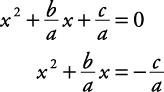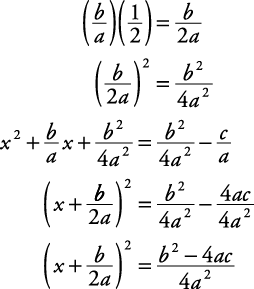The following represents any quadratic equation:

ax 2 + bx + c = 0

This can be solved using the completing the square method to produce a formula that can then be applied to all quadratic equations.

##### Example 1

Solve ax 2 + bx + c = 0, a ≠ 0, for x by using the completing the square method.

ax 2 + bx + c = 0

Get the coefficient of the squared term to be 1.Isolate the variable terms.Complete the square.Apply the square root property.This last result is referred to as the quadratic formula. Remember, the quadratic formula can be used to solve all quadratic equations.##### Example 2

Solve 2 x 2 – 3 x + 4 = 0 by applying the quadratic formula.Note that this is the same problem solved in Example by completing the square. Here, however, it is solved by direct application of the quadratic formula.